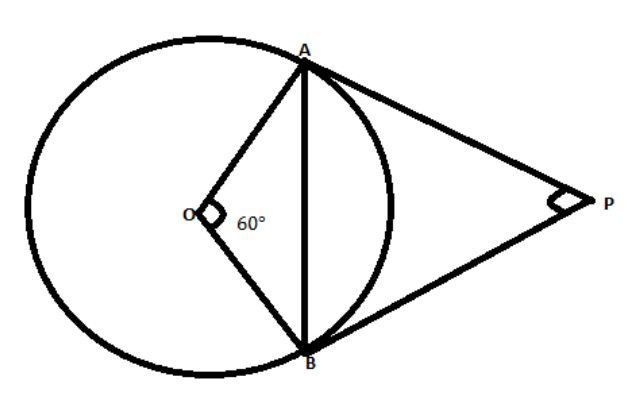QuestionAnswers

# Write true or false and give reasons for your answers.If a chord $AB$ subtends an angle ${60^ \circ }$at the centre of a circle, then then angle between the tangents at $A$ and $B$ is also ${60^ \circ }$.A) TrueB) False

Hint:Using the given information we will try to find the value of the angle between the tangents at $A$ and $B$.We use the property that radius of a circle is perpendicular to the tangent at the tangent point and sum of angles of quadrilaterals is ${360^ \circ }$.Using these properties ,we find the value of angle between tangents. If the value of the angle is ${60^ \circ }$, the statement will be true. Otherwise, it will be false.It is given that: It is given that, $PA$ and $PB$ are tangents to the circle with centre $O$ at $A\,\&\, B,$respectively.
The chord $AB$ subtends an angle ${60^ \circ }$ at $O$.
We have to find the value of the angle $\angle APB = {60^ \circ }$ is true or false.
Now, $PA$ and $PB$ are tangents to the circle with centre $O$ at $A\,\&\, B,$respectively. We know that the radius of a circle is perpendicular to the tangent at the tangent point.
Then, $\angle PAO = \angle PBO = {90^ \circ }$.
By angle sum property we know that, the sum of all the angles of any quadrilaterals is ${360^ \circ }.$
So, from the quadrilateral $APBO$ we get,
$\angle PAO + \angle PBO + \angle AOB + \angle APB = {360^ \circ }$
Substitute the values of the angles we get,
${90^ \circ } + {90^ \circ } + {60^ \circ } + \angle APB = {360^ \circ }$
Simplifying we get,
$\angle APB = {360^ \circ } - {240^ \circ }$
We get, $\angle APB = {120^ \circ }$
So, the given statement is wrong.

So, the correct answer is “Option B”.

Note:The tangent is a straight line which touches any circle at a single point. The radius of a circle is perpendicular to the tangent at the tangent point.By angle sum property we know that, the sum of all the angles of any quadrilateral is ${360^ \circ }$.